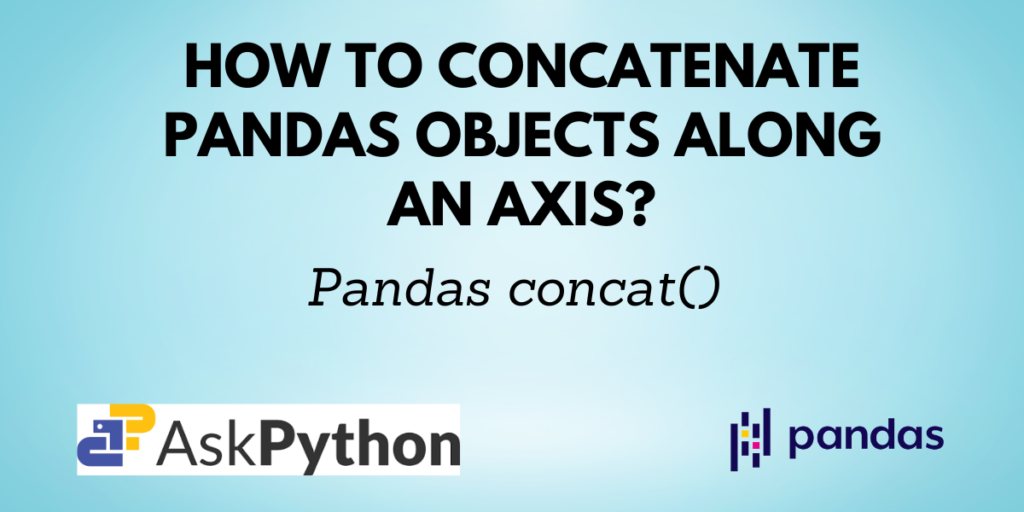# Pandas concat(): Concatenate Pandas objects along a particular axisIn previous tutorials, we’ve covered multiple Pandas methods for reading data, writing data, manipulating data, etc. When it comes to manipulating data, one of the operations performed is joining different data frames. You may need to join data frames along a row or a column or also perform some other manipulation along with it.

The `pandas.concat()` does this job seamlessly. It helps you to concatenate two or more data frames along rows or columns. It creates a new data frame for the result.

In this article, you will learn about the `pandas.concat()` function and also see some examples of how to use it for different purposes.

Also read: Pandas to_excel(): Write an object to an Excel Sheet

## Syntax of Pandas concat()

```pandas.concat(objs, *, axis=0, join='outer', ignore_index=False, keys=None, levels=None, names=None, verify_integrity=False, sort=False, copy=True)
```

Returns: The resulting concatenated object.

Also read: Pandas to_html(): Render a data frame as an HTML table

### Concatenating two data frames with Pandas concat()

Let us first create a data frame.

```import pandas as pd

# creating two dataframes
data1 = {
"Name": ["John", "Charlie"],
"Age": [15, 12]
}

data2 = {
"Name": ["Sarah"],
"Age": 14
}

df1 = pd.DataFrame(data1)
df2 = pd.DataFrame(data2)

print("--- Dataframe 1 ---\n", df1)
print("\n--- Dataframe 2 ---\n", df2)
```

Output:

```--- Dataframe 1 ---
Name  Age
0     John   15
1  Charlie   12

--- Dataframe 2 ---
Name  Age
0  Sarah   14
```

Now, you can concatenate the data frames df1 and df2 using the `concat()` function as follows:

```df = pd.concat([df1, df2])

print("\n--- Concatenated Dataframe  ---\n", df)
```

Output:

```--- Concatenated Dataframe  ---
Name  Age
0     John   15
1  Charlie   12
0    Sarah   14
```

As you can see in the output, the two data frames are concatenated. If you observe the index, you may notice that the indexes are just appended to each other. You can remove this and add an index of the form 0, 1, 2, … n-1 by using the ‘ignore_index‘ parameter.

### Ignoring index

```df = pd.concat([df1, df2], ignore_index=True)

print("\n--- Concatenated Dataframe ---\n", df)
```

Output:

```--- Concatenated Dataframe ---
Name  Age
0     John   15
1  Charlie   12
2    Sarah   14
```

### Sorting the non-concatenation axis

By default, the data frames are concatenated along the rows i.e. axis = 0. So, the default non-concatenation axis is axis = 1 i.e. columns. The ‘sort’ parameter lets you sort the non-concatenation axis as shown below.

```df = pd.concat([df1, df2], sort=True)

print("\n--- Concatenated Dataframe ---\n", df)
```

Output:

```--- Concatenated Dataframe ---
Age     Name
0   15     John
1   12  Charlie
0   14    Sarah
```

Earlier the sequence of columns was Name, Age as the data frames contained the same. Here, the columns are sorted and hence the sequence in the concatenated data frame is changed to Age, Name.

### Concatenation along an axis using Pandas concat()

You can concatenate the data frames along rows or columns.

```# by default, axis=0
df = pd.concat([df1, df2], axis=1)

print("\n--- Concatenated Dataframe ---\n", df)
```

Output:

```--- Concatenated Dataframe ---
Name  Age   Name   Age
0     John   15  Sarah  14.0
1  Charlie   12    NaN   NaN
```

As the code mentioned axis=1, the two data frames were concatenated column-wise. Since the second data frame i.e. df2 contained only one entry, the second entry in the resulting data frames contains missing values i.e. NaN.

### Assigning keys to the concatenated data frame index

```df = pd.concat([df1, df2], keys=['df1', 'df2'])

print("\n--- Concatenated Dataframe ---\n", df)
```

Output:

```--- Concatenated Dataframe ---
Name  Age
df1 0     John   15
1  Charlie   12
df2 0    Sarah   14
```

The output shows the keys for the respective entries in the resulting data frame. These are mostly used to uniquely identify the original data frame that the entry belongs to.

## Summary

`Pandas concat()` is a function in the Pandas library in Python used to concatenate Pandas data frame or series objects. It can concatenate objects along rows or columns.